# The SAEM algorithm for estimating population parameters

$\def\ieta{m} \def\Meta{M} \def\imh{\ell} \def\ceta{\tilde{\eta}} \def\cpsi{\tilde{\psi}} \def\transpose{t} \newcommand{\argmin}{ \mathop{\rm arg} \mathop{\rm min}\limits_{#1} } \newcommand{\argmax}{ \mathop{\rm arg} \mathop{\rm max}\limits_{#1} } \newcommand{\nominal}{#1^{\star}} \newcommand{\psis}{\psi{^\star}} \newcommand{\phis}{\phi{^\star}} \newcommand{\hpsi}{\hat{\psi}} \newcommand{\hphi}{\hat{\phi}} \newcommand{\teps}{\varepsilon} \newcommand{\limite}{\mathop{\longrightarrow}\limits_{\mathrm{#1}}^{\mathrm{#2}}} \newcommand{\DDt}{\partial^2_\theta #1} \def\cpop{c_{\rm pop}} \def\Vpop{V_{\rm pop}} \def\iparam{l} \newcommand{\trcov}{#1} \def\bu{\boldsymbol{u}} \def\bt{\boldsymbol{t}} \def\bT{\boldsymbol{T}} \def\by{\boldsymbol{y}} \def\bx{\boldsymbol{x}} \def\bc{\boldsymbol{c}} \def\bw{\boldsymbol{w}} \def\bz{\boldsymbol{z}} \def\bpsi{\boldsymbol{\psi}} \def\bbeta{\beta} \def\aref{a^\star} \def\kref{k^\star} \def\model{M} \def\hmodel{m} \def\mmodel{\mu} \def\imodel{H} \def\thmle{\hat{\theta}} \def\ofim{I^{\rm obs}} \def\efim{I^{\star}} \def\Imax{\rm Imax} \def\probit{\rm probit} \def\vt{t} \def\id{\rm Id} \def\teta{\tilde{\eta}} \newcommand{\eqdef}{\mathop{=}\limits^{\mathrm{def}}} \newcommand{\deriv}{\frac{d}{dt}#1(t)} \newcommand{\pred}{\tilde{#1}} \def\phis{\phi{^\star}} \def\hphi{\tilde{\phi}} \def\hw{\tilde{w}} \def\hpsi{\tilde{\psi}} \def\hatpsi{\hat{\psi}} \def\hatphi{\hat{\phi}} \def\psis{\psi{^\star}} \def\transy{u} \def\psipop{\psi_{\rm pop}} \newcommand{\psigr}{\hat{\bpsi}_{#1}} \newcommand{\Vgr}{\hat{V}_{#1}} \def\pmacro{\text{p}} \def\py{\pmacro} \def\pt{\pmacro} \def\pc{\pmacro} \def\pu{\pmacro} \def\pyi{\pmacro} \def\pyj{\pmacro} \def\ppsi{\pmacro} \def\ppsii{\pmacro} \def\pcpsith{\pmacro} \def\pcpsiiyi{\pmacro} \def\pth{\pmacro} \def\pypsi{\pmacro} \def\pcypsi{\pmacro} \def\ppsic{\pmacro} \def\pcpsic{\pmacro} \def\pypsic{\pmacro} \def\pypsit{\pmacro} \def\pcypsit{\pmacro} \def\pypsiu{\pmacro} \def\pcypsiu{\pmacro} \def\pypsith{\pmacro} \def\pypsithcut{\pmacro} \def\pypsithc{\pmacro} \def\pcypsiut{\pmacro} \def\pcpsithc{\pmacro} \def\pcthy{\pmacro} \def\pyth{\pmacro} \def\pcpsiy{\pmacro} \def\pz{\pmacro} \def\pw{\pmacro} \def\pcwz{\pmacro} \def\pw{\pmacro} \def\pcyipsii{\pmacro} \def\pyipsii{\pmacro} \def\pcetaiyi{\pmacro} \def\pypsiij{\pmacro} \def\pyipsiONE{\pmacro} \def\ptypsiij{\pmacro} \def\pcyzipsii{\pmacro} \def\pczipsii{\pmacro} \def\pcyizpsii{\pmacro} \def\pcyijzpsii{\pmacro} \def\pcyiONEzpsii{\pmacro} \def\pcypsiz{\pmacro} \def\pccypsiz{\pmacro} \def\pypsiz{\pmacro} \def\pcpsiz{\pmacro} \def\peps{\pmacro} \def\petai{\pmacro} \def\psig{\psi} \def\psigprime{\psig^{\prime}} \def\psigiprime{\psig_i^{\prime}} \def\psigk{\psig^{(k)}} \def\psigki{\psig_i^{(k)}} \def\psigkun{\psig^{(k+1)}} \def\psigkuni{\psig_i^{(k+1)}} \def\psigi{\psig_i} \def\psigil{\psig_{i,\ell}} \def\phig{\phi} \def\phigi{\phig_i} \def\phigil{\phig_{i,\ell}} \def\etagi{\eta_i} \def\IIV{\Omega} \def\thetag{\theta} \def\thetagk{\theta_k} \def\thetagkun{\theta_{k+1}} \def\thetagkunm{\theta_{k-1}} \def\sgk{s_{k}} \def\sgkun{s_{k+1}} \def\yg{y} \def\xg{x} \def\qx{p_x} \def\qy{p_y} \def\qt{p_t} \def\qc{p_c} \def\qu{p_u} \def\qyi{p_{y_i}} \def\qyj{p_{y_j}} \def\qpsi{p_{\psi}} \def\qpsii{p_{\psi_i}} \def\qcpsith{p_{\psi|\theta}} \def\qth{p_{\theta}} \def\qypsi{p_{y,\psi}} \def\qcypsi{p_{y|\psi}} \def\qpsic{p_{\psi,c}} \def\qcpsic{p_{\psi|c}} \def\qypsic{p_{y,\psi,c}} \def\qypsit{p_{y,\psi,t}} \def\qcypsit{p_{y|\psi,t}} \def\qypsiu{p_{y,\psi,u}} \def\qcypsiu{p_{y|\psi,u}} \def\qypsith{p_{y,\psi,\theta}} \def\qypsithcut{p_{y,\psi,\theta,c,u,t}} \def\qypsithc{p_{y,\psi,\theta,c}} \def\qcypsiut{p_{y|\psi,u,t}} \def\qcpsithc{p_{\psi|\theta,c}} \def\qcthy{p_{\theta | y}} \def\qyth{p_{y,\theta}} \def\qcpsiy{p_{\psi|y}} \def\qcpsiiyi{p_{\psi_i|y_i}} \def\qcetaiyi{p_{\eta_i|y_i}} \def\qz{p_z} \def\qw{p_w} \def\qcwz{p_{w|z}} \def\qw{p_w} \def\qcyipsii{p_{y_i|\psi_i}} \def\qyipsii{p_{y_i,\psi_i}} \def\qypsiij{p_{y_{ij}|\psi_{i}}} \def\qyipsi1{p_{y_{i1}|\psi_{i}}} \def\qtypsiij{p_{\transy(y_{ij})|\psi_{i}}} \def\qcyzipsii{p_{z_i,y_i|\psi_i}} \def\qczipsii{p_{z_i|\psi_i}} \def\qcyizpsii{p_{y_i|z_i,\psi_i}} \def\qcyijzpsii{p_{y_{ij}|z_{ij},\psi_i}} \def\qcyi1zpsii{p_{y_{i1}|z_{i1},\psi_i}} \def\qcypsiz{p_{y,\psi|z}} \def\qccypsiz{p_{y|\psi,z}} \def\qypsiz{p_{y,\psi,z}} \def\qcpsiz{p_{\psi|z}} \def\qeps{p_{\teps}} \def\qetai{p_{\eta_i}} \def\neta{n_\eta} \def\ncov{M} \def\npsi{n_\psig} \def\beeta{\eta} \def\logit{\rm logit} \def\transy{u} \def\so{O} \newcommand{\prob}{ \mathbb{P}\left(#1\right)} \newcommand{\probs}{ \mathbb{P}_{#1}\left(#2\right)} \newcommand{\esp}{\mathbb{E}\left(#1\right)} \newcommand{\esps}{\mathbb{E}_{#1}\left(#2\right)} \newcommand{\var}{\mbox{Var}\left(#1\right)} \newcommand{\vars}{\mbox{Var}_{#1}\left(#2\right)} \newcommand{\std}{\mbox{sd}\left(#1\right)} \newcommand{\stds}{\mbox{sd}_{#1}\left(#2\right)} \newcommand{\corr}{\mbox{Corr}\left(#1\right)} \newcommand{\Rset}{\mbox{$\mathbb{R}$}} \newcommand{\Yr}{\mbox{$\mathcal{Y}$}} \newcommand{\teps}{\varepsilon} \newcommand{\like}{\cal L} \newcommand{\logit}{\rm logit} \newcommand{\transy}{u} \newcommand{\repy}{y^{(r)}} \newcommand{\brepy}{\boldsymbol{y}^{(r)}} \newcommand{\vari}{#1_{#2}^{{#3}}} \newcommand{\dA}{\dot{#1}_{#2}(t)} \newcommand{\nitc}{N} \newcommand{\itc}{I} \newcommand{\vl}{V} \newcommand{tstart}{t_{start}} \newcommand{tstop}{t_{stop}} \newcommand{\one}{\mathbb{1}} \newcommand{\hazard}{h} \newcommand{\cumhaz}{H} \newcommand{\std}{\mbox{sd}\left(#1\right)} \newcommand{\eqdef}{\mathop{=}\limits^{\mathrm{def}}} \def\mlxtran{\text{MLXtran}} \def\monolix{\text{Monolix}}$

## Introduction

The SAEM (Stochastic Approximation of EM) algorithm is a stochastic algorithm for calculating the maximum likelihood estimator (MLE) in the quite general setting of incomplete data models. SAEM has been shown to be a very powerful NLMEM tool, known to accurately estimate population parameters as well as having good theoretical properties. In fact, it converges to the MLE under very general hypotheses.

SAEM was first implemented in the $\monolix$ software. It has also been implemented in NONMEM, the R package saemix, and the Matlab statistics toolbox as the function nlmefitsa.m.

Here, we consider a model that includes observations $\by=(y_i , 1\leq i \leq N)$, unobserved individual parameters $\bpsi=(\psi_i , 1\leq i \leq N)$ and a vector of parameters $\theta$. By definition, the maximum likelihood estimator of $\theta$ maximizes

$${\like}(\theta ; \by) = \py(\by ; \theta) = \displaystyle{ \int \pypsi(\by,\bpsi ; \theta) \, d \bpsi}.$$

SAEM is an iterative algorithm that essentially consists of constructing $N$ Markov chains $(\psi_1^{(k)})$, ..., $(\psi_N^{(k)})$ that converge to the conditional distributions $\pmacro(\psi_1|y_1),\ldots , \pmacro(\psi_N|y_N)$, using at each step the complete data $(\by,\bpsi^{(k)})$ to calculate a new parameter vector $\theta_k$. We will present a general description of the algorithm, highlighting the connection with the EM algorithm, and present by way of a simple example how to implement SAEM and use it in practice.

We will also give some extensions of the base algorithm that allow us to improve the convergence properties of the algorithm. For instance, it is possible to stabilize the algorithm's convergence by using several Markov chains per individual. Also, a simulated annealing version of SAEM allows us improve the chances of converging towards the global maximum of the likelihood rather than towards local maxima.

## The EM algorithm

We first remark that if the individual parameters $\bpsi=(\psi_i)$ are observed, estimation is not thwarted by any particular problem because an estimator could be found by directly maximizing the joint distribution $\pypsi(\by,\bpsi ; \theta)$.

However, since the $\psi_i$ are not observed, the EM algorithm replaces $\bpsi$ by its conditional expectation. Then, given some initial value $\theta_0$, iteration $k$ updates ${\theta}_{k-1}$ to ${\theta}_{k}$ with the two following steps:

• $\textbf{E-step:}$ evaluate the quantity

$$Q_k(\theta)=\esp{\log \pmacro(\by,\bpsi;\theta)| \by;\theta_{k-1} } .$$

• $\textbf{M-step:}$ update the estimation of $\theta$:

$$\theta_{k} = \argmax{\theta} \, Q_k(\theta) .$$

In can be proved that each EM iteration increases the likelihood of observations and that the EM sequence $(\theta_k)$ converges to a stationary point of the observed likelihood under mild regularity conditions.

Unfortunately, in the framework of nonlinear mixed-effects models, there is no explicit expression for the E-step since the relationship between observations $\by$ and individual parameters $\bpsi$ is nonlinear. However, even though this expectation cannot be computed in a closed-form, it can be approximated by simulation. For example,

• The Monte Carlo EM (MCEM) algorithm replaces the E-step by a Monte Carlo approximation based on a large number of independent simulations of the non-observed individual parameters $\bpsi$.
• The SAEM algorithm replaces the E-step by a stochastic approximation based on a single simulation of $\bpsi$.

## The SAEM algorithm

At iteration $k$ of SAEM:

• $\textbf{Simulation step}$: for $i=1,2,\ldots, N$, draw $\psi_i^{(k)}$ from the conditional distribution $\pmacro(\psi_i |y_i ;\theta_{k-1})$.

• $\textbf{Stochastic approximation}$: update $Q_k(\theta)$ according to

$$Q_k(\theta) = Q_{k-1}(\theta) + \gamma_k ( \log \pmacro(\by,\bpsi^{(k)};\theta) - Q_{k-1}(\theta) ),$$

where $(\gamma_k)$ is a decreasing sequence of positive numbers such that $\gamma_1=1$, $\sum_{k=1}^{\infty} \gamma_k = \infty$ and $\sum_{k=1}^{\infty} \gamma_k^2 < \infty$.

• $\textbf{Maximization step}$: update $\theta_{k-1}$ according to

$$\theta_{k} = \argmax{\theta} \, Q_k(\theta) .$$

Remarks

• Setting $\gamma_k=1$ for all $k$ means that there is no memory in the stochastic approximation:

$$Q_k(\theta) = \log \pmacro(\by,\bpsi^{(k)};\theta) .$$

This algorithm, known as Stochastic EM (SEM), thus consists of successively simulating $\bpsi^{(k)}$ with the conditional distribution $\pmacro(\bpsi^{(k)} | \by;\theta_{k-1})$, then computing $\theta_k$ by maximizing the joint distribution $\pmacro(\by,\bpsi^{(k)};\theta)$.

• When the number $N$ of subjects is small, convergence of SAEM can be improved by running $L$ Markov chains for each individual instead of one. The simulation step at iteration $k$ then requires us to draw $L$ sequences ${ \phi_i^{(k,1)} } ,\ldots , { \phi_i^{(k,L)} }$ for each individual $i$ and to combine stochastic approximation and Monte Carlo in the approximation step:

$$Q_k(\theta) = Q_{k-1}(\theta) + \gamma_k \left( \frac{1}{L}\sum_{\ell=1}^{L} \log \pmacro(\by,\bpsi^{(k,\ell)};\theta) - Q_{k-1}(\theta) \right) .$$

By default, $\monolix$ selects $L$ so that $N\times L \geq 50$.

Implementation of SAEM is simplified when the complete model $\pmacro(\by,\bpsi;\theta)$ belongs to a regular (curved) exponential family:

$$\pmacro(\by,\bpsi ;\theta) = \exp\left\{ - \zeta(\theta) + \langle \tilde{S}(\by,\bpsi) , \varphi(\theta) \rangle \right\} ,$$

where $\tilde{S}(\by,\bpsi)$ is a sufficient statistics of the complete model (i.e., whose value contains all the information needed to compute any estimate of $\theta$) which takes its values in an open subset ${\cal S}$ of $\Rset^m$. Then, there exists a function $\tilde{\theta}$ such that for any $s\in {\cal S}$,

 $$\tilde{\theta}(s) = \argmax{\theta} \left\{ - \zeta(\theta) + \langle s , \varphi(\theta) \rangle \right\} .$$ (1)

The approximation step of SAEM simplifies to a general Robbins-Monro-type scheme for approximating this conditional expectation:

• $\textbf{Stochastic approximation}$: update $s_k$ according to

$$s_k = s_{k-1} + \gamma_k ( \tilde{S}(\by,\bpsi^{(k)}) - s_{k-1} ) .$$

(Note that the E-step of EM simplifies to computing $s_k=\esp{\tilde{S}(\by,\bpsi) | \by ; \theta_{k-1}}$).

Then, both EM and SAEM algorithms use (1) for the M-step: $\theta_k = \tilde{\theta}(s_k)$.

Precise results for convergence of SAEM were obtained in (???) in the case where $\pmacro(\by,\bpsi;\theta)$ belongs to a regular curved exponential family. This first version of SAEM and these first results assume that the individual parameters are simulated exactly under the conditional distribution at each iteration. Unfortunately, for most nonlinear models or non-Gaussian models, the unobserved data cannot be simulated exactly under this conditional distribution. A well-known alternative consists in using the Metropolis-Hastings algorithm: introduce a transition probability which has as unique invariant distribution the conditional distribution we want to simulate.

In other words, this procedure consists of replacing the Simulation-step of SAEM at iteration $k$ by $m$ iterations of the Metropolis-Hastings (MH) algorithm described in The Metropolis-Hastings algorithm section. It was shown in (???) that SAEM still converges under general conditions when it is coupled with a Markov chain Monte-Carlo procedure.

Remark

Convergence of the Markov chains $(\psi_i^{(k)})$ is not necessary at each SAEM iteration. It suffices to run a few MH iterations with various transition kernels before resetting $\theta_{k-1}$. In $\monolix$ by default three transition kernels are used twice each, successively, in each SAEM iteration.

## Implementing SAEM

Implementation of SAEM can be difficult to describe when looking at complex statistical models such as mixture models, models with inter-occasion variability, etc. We are therefore going to limit ourselves here to looking at some basic models in order to illustrate implementation.

### SAEM for a general hierarchical model

Consider first a very general model for any type (continuous, categorical, survival, etc.) of data $(y_i)$:

$$\begin{eqnarray} y_i | \psi_i &\sim& \pcyipsii(y_i | \psi_i) \\ h(\psi_i) &\sim& {\cal N}( \mu , \Omega), \end{eqnarray}$$

where $h(\psi_i)=(h_1(\psi_{i,1}), h_2(\psi_{i,2}), \ldots , h_d(\psi_{i,d}) )^\transpose$ is a $d$-vector of (transformed) individual parameters, $\mu$ a $d$-vector of fixed effects and $\Omega$ a $d\times d$ variance-covariance matrix.

We assume here that $\Omega$ is positive-definite. Then, a sufficient statistic for the complete model $\pmacro(\by,\bpsi;\theta)$ is $\tilde{S}(\bpsi) = (\tilde{S}_1(\bpsi),\tilde{S}_2(\bpsi))$, where

$$\begin{eqnarray} \tilde{S}_1(\bpsi) &= & \sum_{i=1}^N h(\psi_i) \\ \tilde{S}_2(\bpsi) &= & \sum_{i=1}^N h(\psi_i) h(\psi_i)^\transpose . \end{eqnarray}$$

At iteration $k$ of SAEM, we have:

• $\textbf{Simulation step}$: for $i=1,2,\ldots, N$, draw $\psi_i^{(k)}$ from $m$ iterations of the MH algorithm described in The Metropolis-Hastings algorithm with $\pmacro(\psi_i |y_i ;\mu_{k-1},\Omega_{k-1})$ as limiting distribution.
• $\textbf{Stochastic approximation}$: update $s_k=(s_{k,1},s_{k,2})$ according to

$$\begin{eqnarray} s_{k,1} &=& s_{k-1,1} + \gamma_k \left( \sum_{i=1}^N h(\psi_i^{(k)}) - s_{k-1,1} \right) \\ s_{k,2} &=& s_{k-1,2} + \gamma_k \left( \sum_{i=1}^N h(\psi_i^{(k)})h(\psi_i^{(k)})^\transpose - s_{k-1,2} \right) . \end{eqnarray}$$

• $\textbf{Maximization step}$: update $(\mu_{k-1},\Omega_{k-1})$ according to

$$\begin{eqnarray} \mu_{k} &=& \frac{1}{N} s_{k,1} \\ \Omega_k &=& \frac{1}{N}\left( s_{k,2} - s_{k,1}s_{k,1}^\transpose \right) . \end{eqnarray}$$

What is remarkable is that it suffices to be able to calculate $\pcyipsii(y_i | \psi_i)$ for all $\psi_i$ and $y_i$ in order to be able to run SAEM. In effect, this allows the simulation step to be run using MH since the acceptance probabilities can be calculated.

### SAEM for a continuous data model

Consider now a continuous data model in which the residual error variance is now constant:

$$\begin{eqnarray} y_{ij} &=& f(t_{ij},\phi_i) + a \teps_{ij} \\ h(\psi_i) &\sim& {\cal N}( \mu , \Omega) . \end{eqnarray}$$

Here, the individual parameters are $\psi_i=(\phi_i,a)$. The variance-covariance matrix for $\psi_i$ is not positive-definite in this case because $a$ has no variability. If we suppose that the variance matrix $\Omega$ is positive-definite, then noting $\theta=(\mu,\Omega,a)$, a natural decomposition of the model is:

$$\pmacro(\by,\bpsi;\theta) = \pmacro(\by | \bpsi;a)\pmacro(\bpsi;\mu,\Omega) .$$

The previous statistic $\tilde{S}(\bpsi) = (\tilde{S}_1(\bpsi),\tilde{S}_2(\bpsi))$ is not sufficient for estimating $a$. Indeed, we need an additional component which is function both of $\by$ and $\bpsi$:

$$\tilde{S}_3(\by, \bpsi) =\sum_{i=1}^N \sum_{j=1}^{n_i}(y_{ij} - f(t_{ij},\psi_i))^2.$$

Then,

$$\begin{eqnarray} s_{k,3} &=& s_{k-1,3} + \gamma_k ( \tilde{S}_3(\by, \bpsi) - s_{k-1,3} ) \\ a_k^2 &=& \displaystyle{ \frac{1}{\sum_{i=1}^N n_i} s_{k,3} }\ . \end{eqnarray}$$

The choice of step-size $(\gamma_k)$ is extremely important for ensuring convergence of SAEM. The sequence $(\gamma_k)$ used in \monolix decreases like $k^{-\alpha}$. We recommend using $\alpha=0$ (that is, $\gamma_k=1$) during the first $K_1$ iterations, in order to converge quickly to a neighborhood of a maximum of the likelihood, and $\alpha=1$ during the next $K_2$ iterations. Indeed, the initial guess $\theta_0$ may be far from the maximum likelihood value we are looking for, and the first iterations with $\gamma_k=1$ allow SAEM to converge quickly to a neighborhood of this value. Then, smaller step-sizes ensure the almost sure convergence of the algorithm to the maximum likelihood estimator.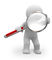Example

Consider a simple model for continuous data:

$$\begin{eqnarray} y_{ij} &\sim& {\cal N}(A_i\,e^{-k_i \, t_{ij} } , a^2) \\ \log(A_i)&\sim&{\cal N}(\log(A_{\rm pop}) , \omega_A^2) \\ \log(k_i)&\sim&{\cal N}(\log(k_{\rm pop}) , \omega_k^2) , \end{eqnarray}$$

where $A_{\rm pop}=6$, $k_{\rm pop}=0.25$, $\omega_A=0.3$, $\omega_k=0.3$ and $a=0.2$. Let us look at the effect of different settings for $(\gamma_k)$ (and $L$) for estimating the population parameters of the model with SAEM.

1. For all $k$, $\gamma_k = 1$: the sequence $(\theta_{k})$ converges very quickly to a neighborhood of the "solution". The sequence $(\theta_{k})$ is a homogeneous Markov Chain that converges in distribution but does not converge almost surely.

2. For all $k$, $\gamma_k = 1/k$: the sequence $(\theta_{k})$ converges almost surely to the maximum likelihood estimate of $\theta$, but very slowly.

3. $\gamma_k = 1$, $k=1$, ...,$40$, $\gamma_k = 1/(k-40)$, $k \geq 41$: the sequence $(\theta_{k})$ converges almost surely to the maximum likelihood estimate of $\theta$, and quickly.

4. $L=10$, $\gamma_k = 1$, $k \geq 1$: the sequence $(\theta_{k})$ is an homogeneous Markov chain that converges in distribution, as in Example 1, but the variance is reduced by a factor $\sqrt{10}$: in this case, SAEM behaves like EM.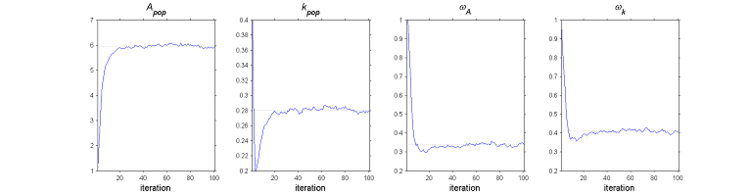## A simple example to understand why SAEM converges in practice

Let us look at a very simple Gaussian model, with only one observation per individual:

$$\begin{eqnarray} \psi_i &\sim& {\cal N}(\theta,\omega^2) , \ \ \ 1 \leq i \leq N \\ y_i &\sim& {\cal N}(\psi_i,\sigma^2) \end{eqnarray}$$

We will furthermore assume that both $\omega^2$ and $\sigma^2$ are known.

Here, the maximum likelihood estimator $\hat{\theta}$ of $\theta$ is easy to compute since $y_i \sim_{i.i.d.} {\cal N}(\theta,\omega^2+\sigma^2)$. We find that

$$\hat{\theta} = \displaystyle{\frac{1}{N} }\sum_{i=1}^{N} y_i .$$

We propose to now try and compute $\hat{\theta}$ using SAEM instead. The simulation step is straightforward since the conditional distribution of $\psi_i$ is a normal distribution:

$$\psi_i | y_i \sim {\cal N}(a \theta + (1-a)y_i , \gamma^2) ,$$

where

$$\begin{eqnarray} a &= & \displaystyle{ \frac{1}{\omega^2} } \left(\displaystyle{ \frac{1}{\sigma^2} }+ \displaystyle{\frac{1}{\omega^2} }\right)^{-1} \\ \gamma^2 &= &\left(\displaystyle{ \frac{1}{\sigma^2} }+ \displaystyle{\frac{1}{\omega^2} }\right)^{-1}. \end{eqnarray}$$

The maximization step is also straightforward. Indeed, a sufficient statistics for estimating $\theta$ is

$${\cal S}(\bpsi) = \sum_{i=1}^{N} \psi_i.$$

Then,

$$\begin{eqnarray} \tilde{\theta}({\cal S(\bpsi)} ) &=& \argmax{\theta} \pmacro(y_1,\ldots,y_N,\psi_1,\ldots,\psi_N;\theta) \\ &=& \argmax{\theta} \pmacro(\psi_1,\ldots,\psi_N;\theta) \\ &=& \frac{ {\cal S}(\bpsi)}{N}. \end{eqnarray}$$

Let us first look at the behavior of SAEM when $\gamma_k=1$. At iteration $k$,

• Simulation step: $\psi_i^{(k)} \sim {\cal N}( a \theta_{k-1} + (1-a)y_i , \gamma^2)$
• Maximization step: $\theta_k = \displaystyle{ \frac{ {\cal S}(\bpsi^{(k)})}{N} } = \displaystyle{ \frac{1}{N} }\sum_{i=1}^{N} \psi_i^{(k)}$.

It can be shown that:

$$\theta_k - \hat{\theta} = a(\theta_{k-1} - \hat{\theta}) + e_k ,$$

where $e_k \sim {\cal N}(0, \gamma^2 /N)$. Then, the sequence $(\theta_k)$ is an autoregressive process of order 1 (AR(1)) which converges in distribution to a normal distribution when $k\to \infty$:

$$\theta_k \limite{}{\cal D} {\cal N}\left(\hat{\theta} , \displaystyle{ \frac{\gamma^2}{N(1-a^2)} }\right) .$$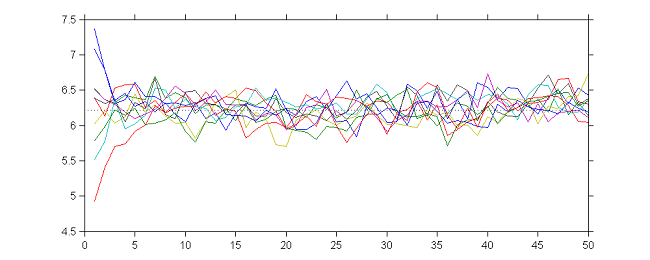10 sequences $(\theta_k)$ obtained with different initial values and $\gamma_k=1$ for $1\leq k \leq 50$

Now, let us see what happens instead when $\gamma_k$ decreases like $1/k$. At iteration $k$,

• Simulation step: $\psi_i^{(k)} \sim {\cal N}( a \theta_{k-1} + (1-a)y_i , \gamma^2)$
• Maximization step:

$$\theta_k = \theta_{k-1} + \displaystyle{ \frac{1}{k} }\left( \displaystyle{ \frac{1}{N} }\sum_{i=1}^{N} \psi_i^{(k)} -\theta_{k-1} \right).$$

Here, we can show that:

$$\theta_k - \hat{\theta} = \displaystyle{ \frac{k-a}{k} }(\theta_{k-1} - \hat{\theta}) + \displaystyle{\frac{e_k}{k} },$$

where $e_k \sim {\cal N}(0, \gamma^2 /N)$. Then, the sequence $(\theta_k)$ converges almost surely to $\hat{\theta}$.

$$\theta_k \limite{}{\rm p.s.} \hat{\theta}$$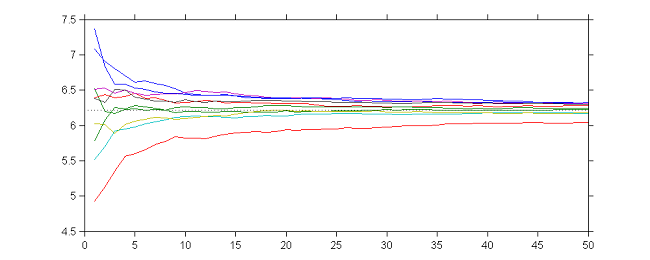10 sequences $(\theta_k)$ obtained with different initial values and $\gamma_k=1/k$ for $1\leq k \leq 50$

Thus, we see that by combining the two strategies, the sequence $(\theta_k)$ is a Markov chain that converges to a random walk around $\hat{\theta}$ during the first $K_1$ iterations, and then converges almost surely to $\hat{\theta}$ during the next $K_2$ iterations.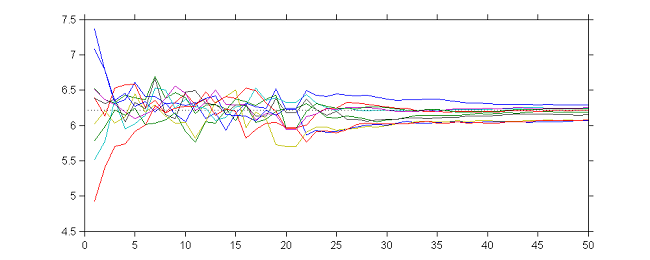10 sequences $(\theta_k)$ obtained with different initial values, $\gamma_k=1$ for $1\leq k \leq 20$ and $\gamma_k=1/(k-20)$ for $21\leq k \leq 50$

 The SAEM algorithm in practice.

## A simulated annealing version of SAEM

Convergence of SAEM can strongly depend on the initial guess if the likelihood ${\like}$ possesses several local maxima. A simulated annealing version of SAEM can improve convergence of the algorithm toward the global maximum of ${\like}$.

To do this, we can first rewrite the joint pdf of $(\by,\bpsi)$ as follows:

$$\begin{eqnarray} \pmacro(\by,\bpsi;\theta) = C(\theta)\, \exp \left\{-U(\by,\bpsi;\theta)\right\} , \end{eqnarray}$$

where $C(\theta)$ is a normalizing constant that only depends on $\theta$.

Then, for any "temperature" $T\geq0$, we consider the complete model

$$\pmacro_T(\by,\bpsi;\theta) = C_T(\theta)\, \exp \left\{-\displaystyle{\frac{1}{T} }U(\by,\bpsi;\theta) \right\} ,$$

where $C_T(\theta)$ is still a normalizing constant.

We introduce a decreasing temperature sequence $(T_k, 1\leq k \leq K)$ and use the SAEM algorithm on the complete model $\pmacro_{T_k}(\by,\bpsi;\theta)$ at iteration $k$ (the usual version of SAEM uses $T_k=1$ at each iteration). The sequence $(T_k)$ is chosen to have large positive values during the first iterations, then decrease with an exponential rate to 1: $T_k = \max(1, \tau \ T_{k-1})$.

Consider for example the following model for continuous data:

$$\begin{eqnarray} y_{ij} &\sim& {\cal N}(f(t_{ij};\psi_i) , a^2) \\ h(\psi_i) &\sim& {\cal N}(\mu , \Omega) . \end{eqnarray}$$

Here, $\theta = (\mu,\Omega,a^2)$ and

$$\pmacro(\by,\bpsi;\theta) = C(\theta)\, \exp \left\{- \displaystyle{ \frac{1}{2 a^2} }\sum_{i=1}^N \sum_{j=1}^{n_i} (y_{ij} - f(t_{ij};\psi_i))^2 - \displaystyle{ \frac{1}{2} } \sum_{i=1}^N (h(\psi_i)-\mu)^\transpose \Omega (h(\psi_i)-\mu) \right\},$$

where $C(\theta)$ is a normalizing constant that only depends on $a$ and $\Omega$.

We see that $\pmacro_T(\by,\bpsi;\theta)$ will also be a normal distribution whose residual error variance $a^2$ is replaced by $T a^2$ and variance matrix of the random effects $\Omega$ by $T\Omega$. In other words, a model with a "large temperature" is a model with large variances.

The algorithm therefore consists in choosing large initial variances $\Omega_0$ and $a^2_0$ (that include the initial temperature $T_0$ implicitly) and setting $a^2_k = \max(\tau \ a^2_{k-1} , \hat{a}(\by,\bpsi^{(k)})$ and $\Omega_k = \max(\tau \ \Omega_{k-1} , \hat{\Omega}(\bpsi^{(k)})$ during the first iterations of the algorithm. Here, $0\leq\tau\leq 1$.

These large values of the variance make the conditional distributions $\pmacro_T(\psi_i | y_i;\theta)$ less concentrated around their modes. This procedure thus allows the sequence $(\theta_k)$ to "escape" from local maxima of the likelihood during the first iterations of SAEM and to converge to a neighborhood of the global maximum of ${\like}$. After these initial iterations, the usual SAEM algorithm is used to estimate these variances at each iteration.

Remark

We can use two different coefficients $\tau_1$ and $\tau_2$ for $\Omega$ and $a^2$ in $\monolix$. It is possible, for example, to choose $\tau_1<1$ and $\tau_2>1$, with large initial inter-subject variances $\Omega_0$ and small initial residual variance $a^2_0$. In this case, SAEM tries to obtain the best possible fit during the first iterations, allowing a large inter-subject variability. During the next iterations, this variability is reduced and the residual variance increases until reaching the best possible trade-off between these two criteria.A PK example

Consider a simple one-compartment model for oral administration:
 $$f(t;ka,V,k) = \displaystyle{ \frac{D\, ka}{V(ka-ke)} }\left( e^{-ke \, t} - e^{-ka \, t} \right) .$$ (2)

We then simulate PK data from 80 patients using the following population PK parameters:

$$ka_{\rm pop} = 1, \quad V_{\rm pop}=8, \quad ke_{\rm pop}=0.25 .$$

We can see that the following parametrization gives the same prediction as the one given in (2):

$$\tilde{ka} = ke, \quad \tilde{V}=V \times ke/ka, \quad \tilde{ke}=ka .$$

We can then expect a (global) maximum around $(ka,V,ke) = (1, \ 8, \ 0.25)$ and a (local) maximum of the likelihood around $(ka,V,ke) = (0.25, \ 2, \ 1)$.

The figure below displays the convergence of SAEM without simulated annealing to a local maximum of the likelihood (deviance = $-2\,\log {\like}) =816$). The initial values of the population parameters we chose were $(ka_0,V_0,k_0) = (1,1,1)$.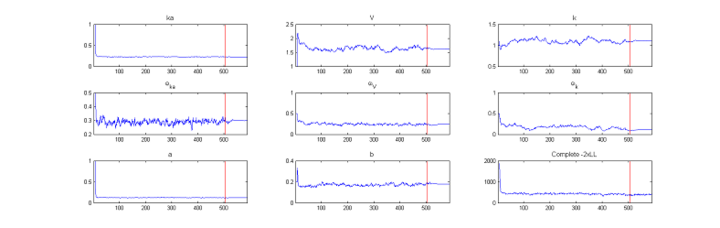Convergence of SAEM to a local maxima of the likelihood

Using the same initial guess, the Simulated Annealing version of SAEM converges to the global maximum of the likelihhod (deviance = 734).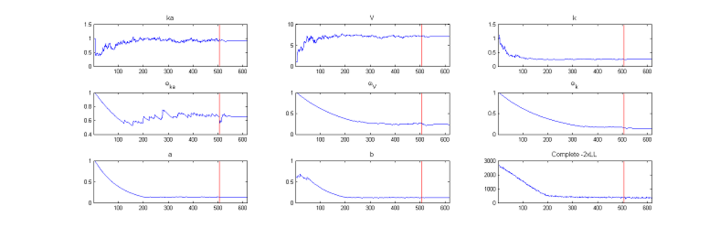Convergence of SAEM to the global maxima of the likelihood